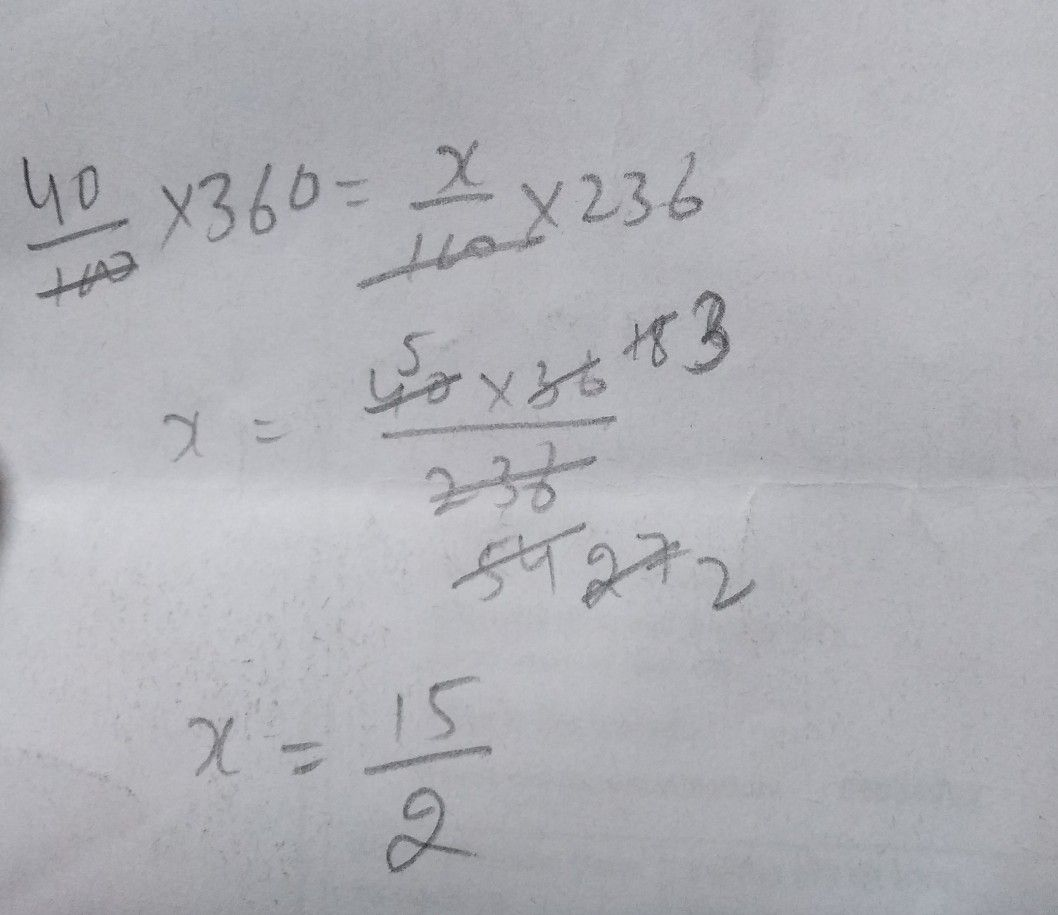Symbol
Problem$4O/^{0}7$ $0$ $0$ $360=x/920$ $0$
10th-13th grade
Other
Question content
40% of 360=x% of 236
SolutionQanda teacher - PARVEEN KUStudent
Thanks a lot for the reply
Thanks for solving my problemsQanda teacher - PARVEEN KU
rate and comment